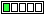All about flooble | fun stuff | Get a free chatterbox | Free JavaScript | Avatarsperplexus dot infoDon't be confused (Posted on 2016-11-14)If x satisfy the equation x^4 - x^2 + 1 = 0 , find the value of x^5 + 1/x

 No Solution Yet Submitted by Danish Ahmed Khan No RatingComments: ( Back to comment list | You must be logged in to post comments.)Solution| Comment 2 of 4 |Multiply both sides of x^4 - x^2 + 1 = 0 by (x^2 + 1) to get x^6 + 1 = 0.

Then rearrange x^6 + 1 = 0 into x^5 = -1/x.

Then x^5 + 1/x = -1/x + 1/x = 0.

 Posted by Brian Smith on 2016-11-14 11:13:44Please log in:

 Search: Search body:
Forums (1)